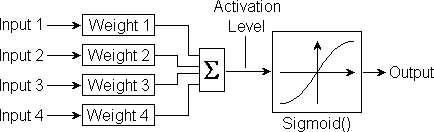Neural Networks: The Completed Neuron

Introduction, input summation, sigmoid function, the complete neuron, testing.

Introduction

The complete neuron comprises the weighted inputs summation function and the sigmoid transfer function as shown below:Each input is multiplied by a corresponding weight. All the products are then added together and divided by the number of inputs to yield what is termed the 'acti­vation level'. This is then fed in as input to the Sigmoid() function to produce the neuron's output.

Input Summation

In the document wsum.htm and associated source files, we developed the optimum 'C' code for computing a neuron's activation level from its inputs and their corres­ponding weights. This code is shown below:

int i, al, *pi, *pw;
long x, Hi, Lo;
for(pi = I, pw = W, Hi = 0, Lo = 0, i = 0; i < NI; i++) {
x = (long)*(pi + i) * *(pw + i);
Lo += x & 0xFFFF;
Hi += x >> 16;
}
al = ((Hi << 1) + (Lo >> 15)) / NI;

Sigmoid Function

Then, in the document SIGMOID.HTM and associated source files, we developed the optimum 'C' source code for the Sigmoid() function as shown below:

int Sigmoid(int x) {
int s, y, j;
if((s = x) < 0) x = -x;
y = *(SigTab + (j = x >> 5));
y += ((*(SigTab + j + 1) - y) * (x & 0x1F)) >> 5;
if (s < 0) y = -y;
return(y);
}

The Complete neuron

We will now combine these two separately developed and tested pieces of code to form a function to simulate a complete neuron:

int Neuron(int *pi, int *pw, int NI) {
register i;      // input array index, sigmoid table index
int a, o, s;     // activation level, neuron output, sign
long P, Hi, Lo;  // long product, high & low accumulators

for(Hi = 0, Lo = 0, i = 0; i < NI; i++) {
P = (long)*(pi + i) * *(pw + i);
Hi += P >> 16;
Lo += P & 0xFFFF;
}
if((s = (a = ((Hi << 1) + (Lo >> 15)) / NI)) < 0)
a = -a;
o = *(SigTab + (i = a >> 5));
o += ((*(SigTab + i + 1) - o) * (a & 0x1F)) >> 5;
if (s < 0) o = -o;
return(o);
}

Note that the names of some of the variables have been changed in order to ration­alise them and help to identify better what they do. The comments adjacent to their declarations explain the new names. We have assigned the index variable 'i' to a register in an attempt to increase speed further.

Testing

The following exerciser was then written and used to test the completed neuron function:

#include <stdio.h>
#define R  32767
#define RR 65556  // 65534 + 22
#define NI    77  // number of inputs to the neuron
int AL;
short	SigTab;

int I[] = {	 // inputs
11376, 13425, 17920, 30226, 28763, 18940, 15329,
11376, 13425, 17920, 30226, 28763, 18940, 15329,
11376, 13425, 17920, 30226, 28763, 18940, 15329,
11376, 13425, 17920, 30226, 28763, 18940, 15329,
11376, 13425, 17920, 30226, 28763, 18940, 15329,
11376, 13425, 17920, 30226, 28763, 18940, 15329,
11376, 13425, 17920, 30226, 28763, 18940, 15329,
11376, 13425, 17920, 30226, 28763, 18940, 15329,
11376, 13425, 17920, 30226, 28763, 18940, 15329,
11376, 13425, 17920, 30226, 28763, 18940, 15329,
11376, 13425, 17920, 30226, 28763, 18940, 15329
};

int W[] = {	 // weights
12345, 21345, 31245, 16730, 31662, 25460, 13557,
12345, 21345, 31245, 16730, 31662, 25460, 13557,
12345, 21345, 31245, 16730, 31662, 25460, 13557,
12345, 21345, 31245, 16730, 31662, 25460, 13557,
12345, 21345, 31245, 16730, 31662, 25460, 13557,
12345, 21345, 31245, 16730, 31662, 25460, 13557,
12345, 21345, 31245, 16730, 31662, 25460, 13557,
12345, 21345, 31245, 16730, 31662, 25460, 13557,
12345, 21345, 31245, 16730, 31662, 25460, 13557,
12345, 21345, 31245, 16730, 31662, 25460, 13557,
12345, 21345, 31245, 16730, 31662, 25460, 13557
};

void SigGen(void) {
int i;
for(i = 0; i < 1024; i++)
SigTab[i] = (double)(
RR /(1 + exp(-((double)(((long)(i)) << 8))/R))- R
);
SigTab = R;
}

int Neuron(int *pi, int *pw, int ni) {
register i;      // input array index, sigmoid table index
int a, o, s;     // activation level, output, sign
long P, Hi, Lo;  // long product, high & low accumulators

for(Hi = 0, Lo = 0, i = 0; i < ni; i++) {
P = (long)*(pi + i) * *(pw + i);
Hi += P >> 16;
Lo += P & 0xFFFF;
}
if((s = (a = ((Hi << 1) + (Lo >> 15)) / ni)) < 0) a = -a;
o = *(SigTab + (i = a >> 5));
o += ((*(SigTab + i + 1) - o) * (a & 0x1F)) >> 5;
if (s < 0) o = -o;
AL = a;          // extra line to check activation level
return(o);
}

main() {
int OP;
SigGen();
OP = Neuron(I, W, NI);
printf("Activation Level = %6d\n", AL);
printf("Neuron Output    = %6d\n", OP);
}

The results displayed by this exerciser are as follows:

Activation Level = 13485
Neuron Output    = 30439

The neuron function can now be used to build into a complete multi-layer percep­tron. This we do in the document mlpc.htm.

© March 1993 Robert John Morton